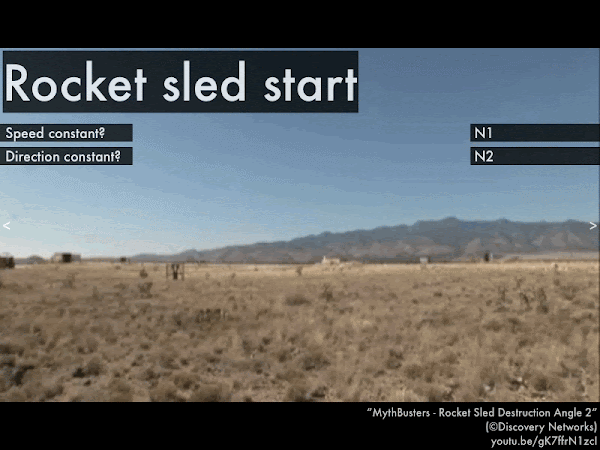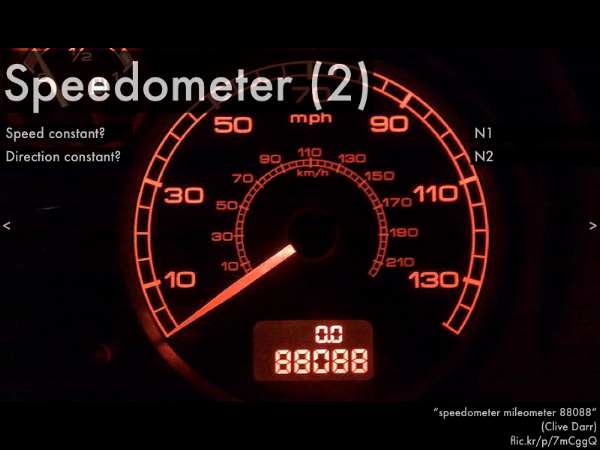## 20180910

### Online reading assignment: forces and motion

Physics 205A, fall semester 2018
Cuesta College, San Luis Obispo, CA

Students have a bi-weekly online reading assignment (hosted by SurveyMonkey.com), where they answer questions based on reading their textbook, material covered in previous lectures, opinion questions, and/or asking (anonymous) questions or making (anonymous) comments. Full credit is given for completing the online reading assignment before next week's lecture, regardless if whether their answers are correct/incorrect. Selected results/questions/comments are addressed by the instructor at the start of the following lecture.

The following questions were asked on reading textbook chapters and previewing a presentation on forces and motion.Selected/edited responses are given below.

Describe what you understand from the assigned textbook reading or presentation preview. Your description (2-3 sentences) should specifically demonstrate your level of understanding.
"As long as speed and direction are constant, Newton's first law applies. When a net force acts upon an object and isn't zero, Newton's second law applies."

"The presentation discusses how to differentiate between Newton's first and second law. The first law applies to objects with constant speed and direction, and the second law applies to objects without constant speed or constant direction."

"The motion flowchart from the presentations helped me simplify how distinguish between Newton's first and second law easier. If the speed and direction is constant then Newton's first law applies, but if they are not then Newton's second law applies."

"Newton's laws will take some more work for me. But from my initial understanding, Newton's first law says that both direction and speed are constant the forces will cancel and there is no change in motion. The second law says that speed and/or direction changes, then the acceleration is non-zero, and the net force must be non-zero."

"When to apply Newton's first and second law. However I am still slightly confused about exactly when to because at times it's kind of a guess."

"I don't understand anything."

Describe what you found confusing from the assigned textbook reading or presentation preview. Your description (2-3 sentences) should specifically identify the concept(s) that you do not understand.
"What the heck classifies something as 'constant?' If an object is not moving does it have a constant speed/direction? If it starts moving after the question is asked like the (rocket sled) is the speed constant? Why are there two components (x and y) to net force? "

"I'm still just a little confused about the definitions of the two laws and how they relate to net forces."

"Can you give us real life examples of each of Newton's laws for a better understanding?"

"I am not exactly sure how to decide if the net force is zero or non-zero. I do understand that equal but opposite forces can cancel each other out, but what about others?"

"Determining whether something has zero or non-zero net force."

"All of it because I haven’t read :("

Identify whether a zero or non-zero net force corresponds to Newton's first law or Newton's second law. (Only correct responses shown.)
Zero net force (ΣF = 0): Newton's first law ("N1") [89%]
Non-zero net force (ΣF ≠ 0): Newton's second law ("N2") [86%]For the rocket sled, Newton's __________ law applies to the motion of this object, and the forces acting on the object add up to a __________ net force.
 first; zero. ************  second; non-zero. ****************************  (Unsure/guessing/lost/help!) ****While the F-35B is descending at a steady rate (before it touches the ground), Newton's __________ law applies to the motion of this object, and the forces acting on the object add up to a __________ net force.
 first; zero. ***************************  second; non-zero. ***********  (Unsure/guessing/lost/help!) ******For this car with a steady speedometer reading (and assuming the shaking is due to the person holding the camera, and not from moving the car itself), Newton's __________ law applies to the motion of this object, and the forces acting on the object add up to a __________ net force.
 first; zero. ********************************  second; non-zero. *****  (Unsure/guessing/lost/help!) *******For a person in the swinging chair ride moving along a circular trajectory at a constant speed, Newton's __________ law applies to the motion of this object, and the forces acting on the object add up to a __________ net force.
 first; zero. ********************  second; non-zero. ******************  (Unsure/guessing/lost/help!) ******For this car with a zero speedometer reading, Newton's __________ law applies to the motion of this object, and the forces acting on the object add up to a __________ net force.
 first; zero. *******************************  second; non-zero. ****  (Unsure/guessing/lost/help!) ****** 

Ask the instructor an anonymous question, or make a comment. Selected questions/comments may be discussed in class.
"If we could go over some of the harder problems, so that they become easier, that would be great."

"Should I already know how to classify things as constant motion or not? Because I feel like this section really assumed that I knew this already." (Well, at least attempting to classify these examples is a start. We'll go over these in more detail in class.)

"Just for clarification the question about the speedometer of a car going 0 mph, Newton's first law will apply not only to the motion of the car, but also the forces acting on the car as well, right? Since the car is not moving, then the net force is zero, and Newtons first law should apply?" (Yes.)

"In the book it never had wording such as zero and non-zero net force. Newton's first law states that an object will stay in motion (with a constant speed and direction) or in rest unless compelled to change its state by a net force. The purpose of a net force is to change the object's velocity but at rest and in a constant velocity neither one requires a net force to sustain it. So would that mean that the net force is zero for objects in rest or in a constant velocity?" (Yes.)

"The examples in the presentation weren't extremely clear with the actual forces involved and the examples don't really say if the speed is constant or not, so it was kind of a guess."

"Can we go over these in class?"

"I would like to talk more about net force."

"May the force be with you."

"I think the class would benefit from more midterm prep. This is off-topic but I saw the 'extraterrestrial hypothesis' post on your blog and was wondering if you are an ET enthusiast? " (The class just before the midterm later this semester is dedicated to review. As for the extraterrestrial hypothesis (the possibility of life elsewhere in the Milky Way galaxy), I feel that it might be true--not a strong hunch, I'm kinda "meh" about it.)